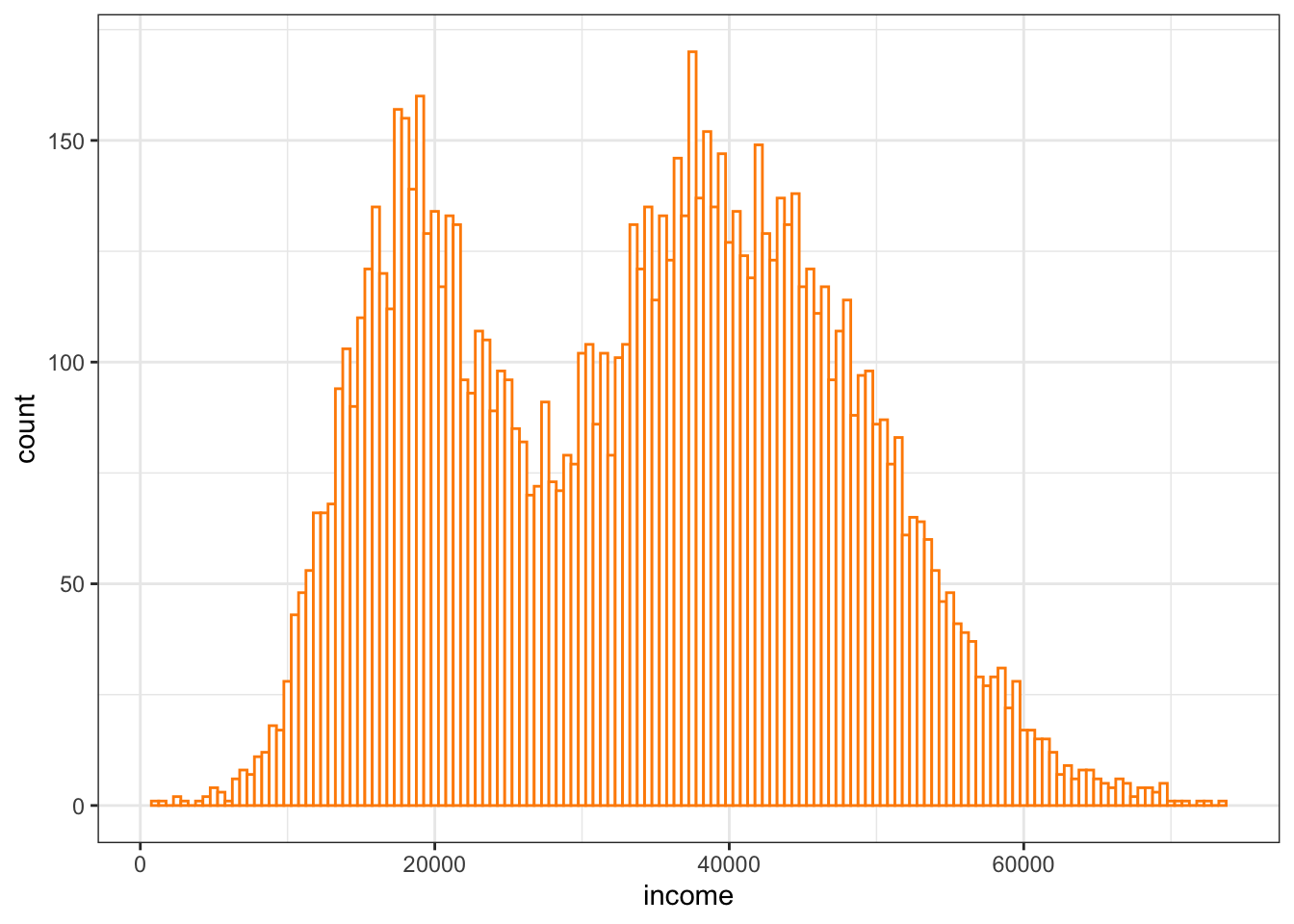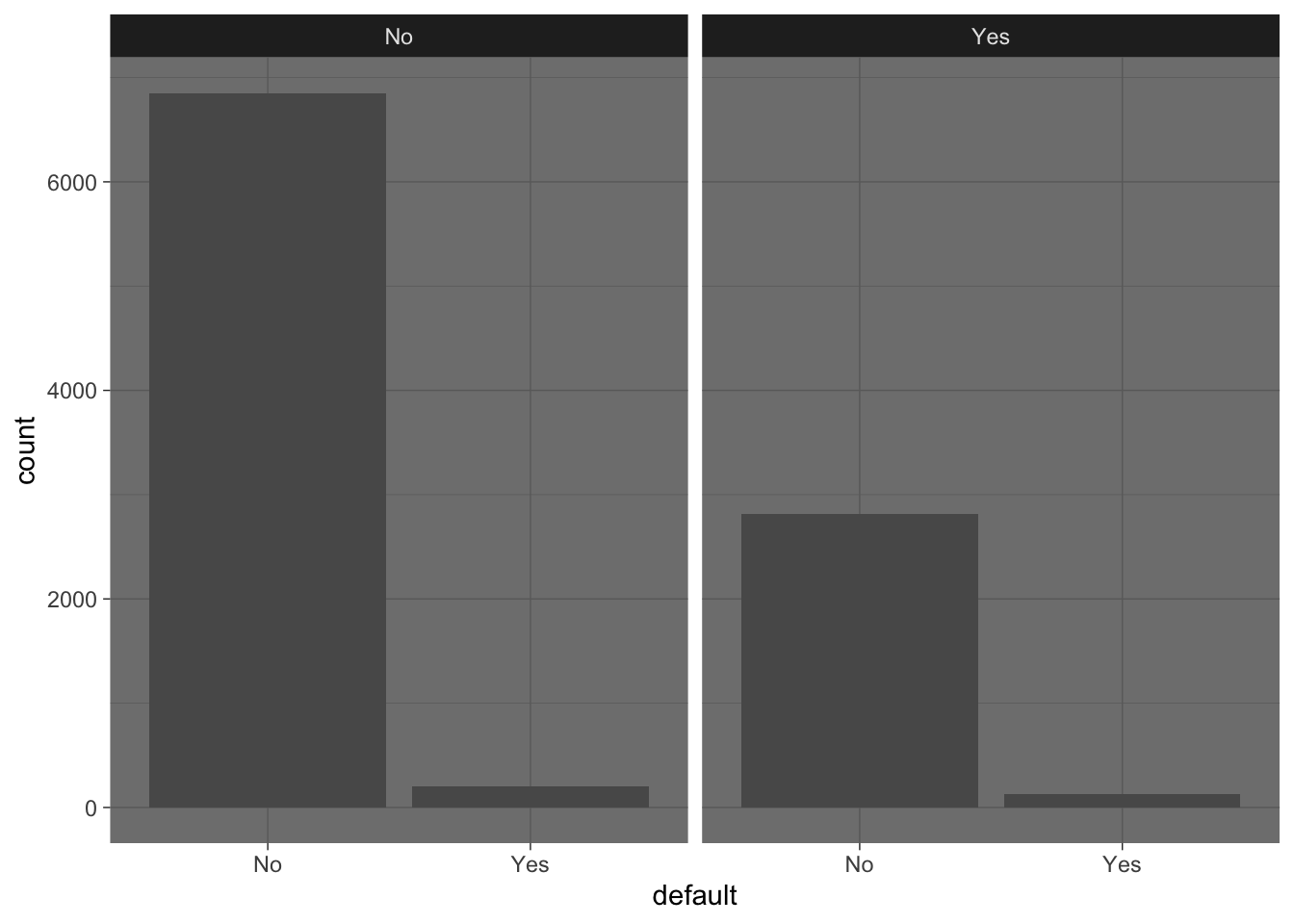# Logistic regression application: credit default

The aim is to predict whether a client will have a credit card default from a few simple covariates.

In the ISLR package, there is a dataset Default which measures for 10000 clients 4 variables: - default: a factor variable corresponding to the presence and the absence of default - student: a factor variable having value Yes for a student and No otherwise - balance: the average credit card balance at the end of the month - income : the customer income

Load the Default data set in the package ISLR. Can you describe the data? If you want to predict the credit default, what will be the outcome?

Solution 1

``````library(ISLR)
data(Default)

10000 observations, 4 columns, the outcome will be default (a binary covariate)

End of solution 1

## Question 2: data exploration

Perform typical univariate and bivariate data exploration. Can you already observe a trend?

Solution 2

Here you can find several plots with sometimes strange color. It is to show you different parameters to tune and choose your favorite plots. But don’t forget you can find a lot of examples online.

``library(ggplot2)``
``## Warning: package 'ggplot2' was built under R version 3.6.2``
``````ggplot(Default, aes(x = income)) +
geom_histogram(binwidth = 500, fill = "white", color = "darkorange") +
theme_bw()````````````ggplot(Default, aes(x = default)) +
geom_bar() +
facet_grid(~student) +
theme_dark()````````````ggplot(Default, aes(x = default, y = income)) +
geom_violin() +
theme_bw() +
geom_jitter(alpha = 0.05)``````